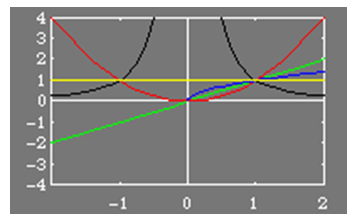Graphs

Recall from the previous lesson, that the family of functions known as the power functions take the form, y = kxp. The shape of the graph of these power functions depends on the sign of k and the value of p. The coefficient k will change the scale of the graph and, if negative, flip the graph across the x-axis.

All graphs of y = xp pass through the point (1,1). Beyond that, the shape and growth for large positive x is given by:

· p >1: resembles a quadratic when even or a cubic when odd. Grows as x grows larger. Example: y = x2

· p = 1: the line y = x. Grows as x grows larger. Example: y = x

· 0 < p <1: concave down. Grows as x grows larger. Example: y = x(½)

· p = 0: the line y = 1. Example: y = x0 = 1

· p < 0: concave up. Approaches 0 as x grows larger. Example: y = x-2